Thermodynamics and Propulsion

# 17.3 Dimensionless Numbers and Analysis of Results

Phenomena in fluid flow and heat transfer depend on dimensionless parameters. The Mach number and the Reynolds number are two you have already seen. These parameters give information as to the relevant flow regimes of a given solution. Casting equations in dimensionless form helps show the generality of application to a broad class of situations (rather than just one set of dimensional parameters). It is generally good practice to use non-dimensional numbers, forms of equations, and results presentation whenever possible. The results for heat transfer from the cylinder are already in dimensionless form but we can carry the idea even further. For the cylinder, we had in Equation (17.25),The parameter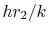or, whereis a relevant length for the particular problem of interest, is called the Biot number, denoted by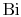. In terms of this parameter,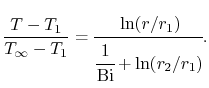(17..29)

The size of the Biot number gives a key to the regimes in which different features are dominant. Forthe convection heat transfer process offers little resistance to heat transfer. There is thus only a small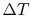outside (i.e.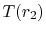close to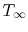) compared to thethrough the solid with a limiting behavior ofasgoes to infinity. This is much like the situation with an external temperature specified.

Forthe conduction heat transfer process offers little resistance to heat transfer. The temperature difference in the body (i.e. from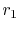to) is small compared to the external temperature difference,. In this situation, the limiting case isIn this regime there is approximately uniform temperature in the cylinder. The size of the Biot number thus indicates the regimes where the different effects become important.

Figure 17.9 shows the general effect of Biot number on temperature distribution. Figure 17.10 is a plot of the temperature distribution in the cylinder for values of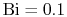,and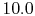.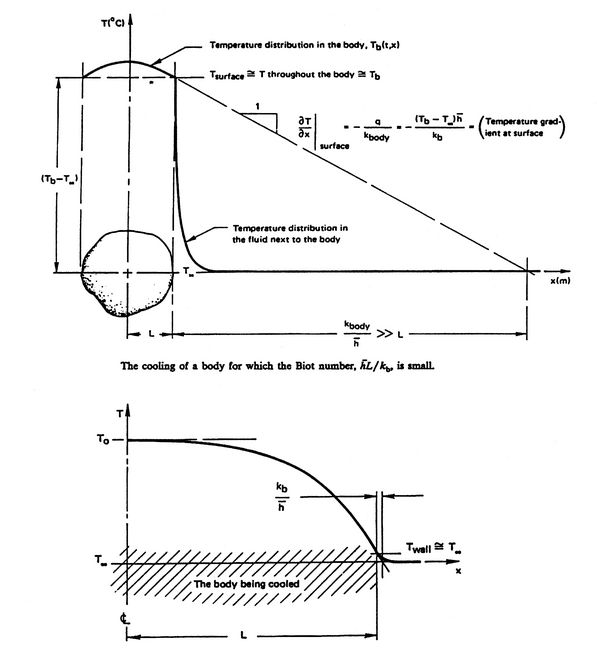UnifiedTP